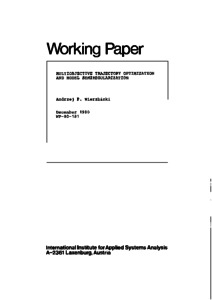# Multiobjective Trajectory Optimization and Model Semiregularization

Wierzbicki, A.P. (1980). Multiobjective Trajectory Optimization and Model Semiregularization. IIASA Working Paper. IIASA, Laxenburg, Austria: WP-80-181Preview Text WP-80-181.pdf Download (1MB) | Preview

## Abstract

The typical formulation of an optimal control or dynamic optimization problem is to optimize a scalar performance functional; less frequently, also vectors of performance functionals are considered in multiobjective optimization. However, there are practical problems -- mostly related to the use of dynamic control models in economic planning -- where the objectives are stated in terms of desirable trajectories. If the goal would be to approximate the desired trajectory from both sides, then the problem could be equivalently stated as a typical approximation problem. However, in many cases the desired trajectories have the meaning of aspiration levels: if possible, they should be exceeded.

The paper presents a mathematical formulation of a multiobjective trajectory optimization problem, various theoretical approaches to this problem -- including interpretations as a generalized Lagrangian functional approach and as a semi-regularization procedure for ill-posed problems, a review of possible computational approaches and examples of actual computations.

Item Type: Monograph (IIASA Working Paper) System and Decision Sciences - Core (SDS) IIASA Import 15 Jan 2016 01:47 27 Aug 2021 17:09 https://pure.iiasa.ac.at/1288View Item